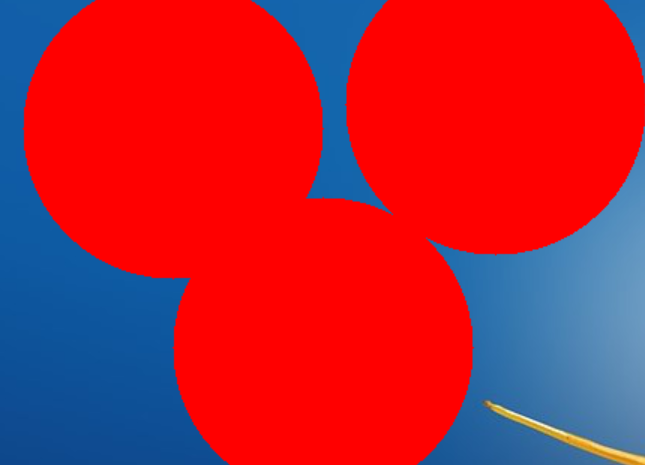# Drawing with Mouse on Images using Python-OpenCV

OpenCV is a huge open-source library for computer vision, machine learning, and image processing. OpenCV supports a wide variety of programming languages like Python, C++, Java, etc. It can process images and videos to identify objects, faces, or even the handwriting of a human. In this article, we will try to draw on images with the help of the mouse. Before learning how to draw on images using a mouse, we need to understand what is a callback.<

### Callback

A callback in programming means to call this function (the callback) when a process completes. The same applies to event-oriented programming in general. When a mouse button is clicked (an event), call a function. We don’t know when the button will be clicked. All we can do is tell the button to “call me back” or call this function when the mouse button is clicked.

### Mouse Callbacks

A callback can happen when a user performs an operation using the mouse; this operation is usually known as an event. Only one callback is present for a mouse, which is setMouseCallback(), all mouse operation will call this function only.

We can have conditional blocks to execute something based on the event/operation performed using the mouse. The mouse events/operations could be:

• EVENT_MOUSEMOVE
• EVENT_LBUTTONDOWN
• EVENT_RBUTTONDOWN
• EVENT_LBUTTONUP
• EVENT_RBUTTONUP

When should this callback occur :

We want to have this call back only when we use the mouse on the pop-up window, which has the title as “Title of Popup Window.”

```cv2.namedWindow("Title of Popup Window")
```

Example 1: Draw Circle when we left-click on a popup with OpenCV :

 `import` `cv2 ` ` `  ` `  `img ``=` `cv2.imread(``"flower.jpg"``) ` ` `  `def` `draw_circle(event, x, y, flags, param): ` `     `  `    ``if` `event ``=``=` `cv2.EVENT_LBUTTONDOWN: ` `        ``print``(``"hello"``) ` `        ``cv2.circle(img, (x, y), ``100``, (``0``, ``255``, ``0``), ``-``1``) ` `         `  `cv2.namedWindow(winname ``=` `"Title of Popup Window"``) ` `cv2.setMouseCallback(``"Title of Popup Window"``, draw_circle) ` ` `  `while` `True``: ` `    ``cv2.imshow(``"Title of Popup Window"``, img) ` `     `  `    ``if` `cv2.waitKey(``10``) & ``0xFF` `=``=` `27``: ` `        ``break` `         `  `cv2.destroyAllWindows() `

Output:Example 2: Drawing a Rectangle by dragging on Images with OpenCV

 `import` `cv2 ` ` `  ` `  `img ``=` `cv2.imread(``"flower.jpg"``) ` ` `  `# variables ` `ix ``=` `-``1` `iy ``=` `-``1` `drawing ``=` `False` ` `  `def` `draw_rectangle_with_drag(event, x, y, flags, param): ` `     `  `    ``global` `ix, iy, drawing, img ` `     `  `    ``if` `event ``=``=` `cv2.EVENT_LBUTTONDOWN: ` `        ``drawing ``=` `True` `        ``ix ``=` `x ` `        ``iy ``=` `y             ` `             `  `    ``elif` `event ``=``=` `cv2.EVENT_MOUSEMOVE: ` `        ``if` `drawing ``=``=` `True``: ` `            ``cv2.rectangle(img, pt1 ``=``(ix, iy), ` `                          ``pt2 ``=``(x, y), ` `                          ``color ``=``(``0``, ``255``, ``255``), ` `                          ``thickness ``=``-``1``) ` `     `  `    ``elif` `event ``=``=` `cv2.EVENT_LBUTTONUP: ` `        ``drawing ``=` `False` `        ``cv2.rectangle(img, pt1 ``=``(ix, iy), ` `                      ``pt2 ``=``(x, y), ` `                      ``color ``=``(``0``, ``255``, ``255``), ` `                      ``thickness ``=``-``1``) ` `         `  `cv2.namedWindow(winname ``=` `"Title of Popup Window"``) ` `cv2.setMouseCallback(``"Title of Popup Window"``,  ` `                     ``draw_rectangle_with_drag) ` ` `  `while` `True``: ` `    ``cv2.imshow(``"Title of Popup Window"``, img) ` `     `  `    ``if` `cv2.waitKey(``10``) ``=``=` `27``: ` `        ``break` ` `  `cv2.destroyAllWindows() `

Output:### What does (cv2.waitKey(10) & 0xFF == 27) do ?

cv2.waitKey() returns a 32 Bit integer value (might be dependent on the platform). The key input is in ASCII which is an 8 Bit integer value. So you only care about these 8 bits and want all other bits to be 0. This you can achieve with: `cv2.waitKey(10) & 0xFF == 27`

My Personal Notes arrow_drop_upCheck out this Author's contributed articles.

If you like GeeksforGeeks and would like to contribute, you can also write an article using contribute.geeksforgeeks.org or mail your article to contribute@geeksforgeeks.org. See your article appearing on the GeeksforGeeks main page and help other Geeks.

Please Improve this article if you find anything incorrect by clicking on the "Improve Article" button below.

Article Tags :

Be the First to upvote.

Please write to us at contribute@geeksforgeeks.org to report any issue with the above content.